Like   Tweet   Pin   +1

 /* styles */ Hi Everyone, after a U.K. heatwave and hottest summer solstice for over 40 years here's news of a series of exhibitions featuring some cool shadows.

## ‘Shadows of the Past‘, London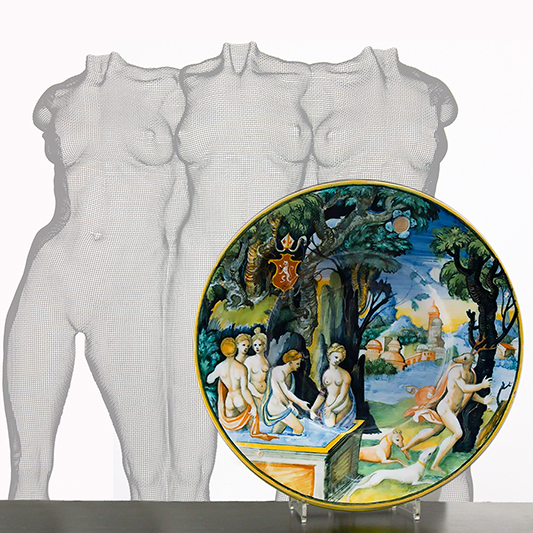Triple composition ‘PSYNTIMATES‘ and ‘Nordi‘ Dish, tin-glazed earthenware, circa 1535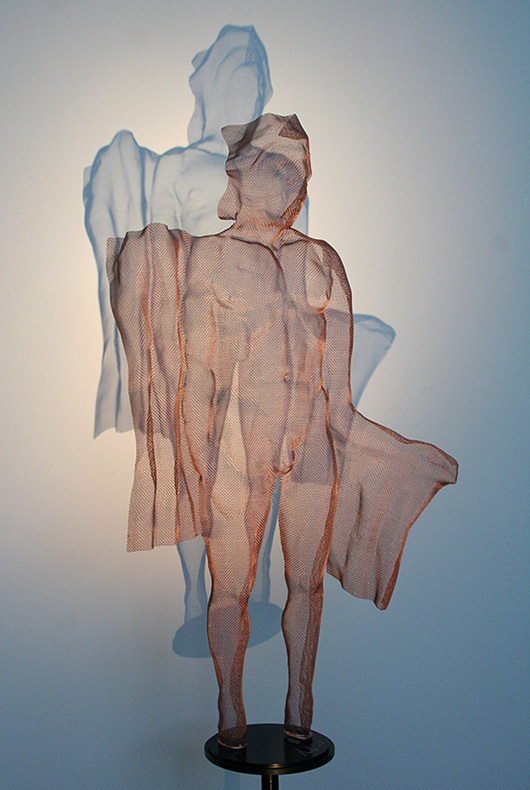‘MYTHOS I‘, steelmesh, 2017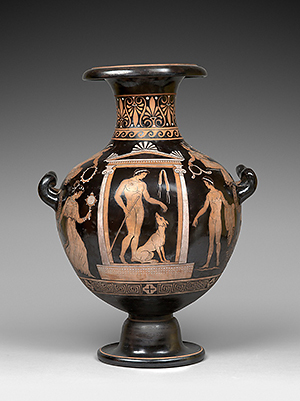Large Etruscan type vase
 /* styles */ ContiniArtUK 105 New Bond Street, London 30th June – 7th July 2017. I'd like to invite you to the forthcoming collaborative exhibition at ContiniArtUK. Many thanks to Cristian Contini and Justin Raccanello from Bazaart for making this exhibition possible. ‘Shadows of the Past‘ is an exciting and very unusual collaboration for ‘London Art Week‘ combining artifacts from the past with contemporary sculpture. Camille Leprince and Justin Raccanello's choice is highly selective for quality, rarity and condition of each ceramic on show. I have created new sculpture (see banner above, detail of ‘BATHERS‘ 2017). for this joint exhibition inspired by a number of the renaissance ceramics presented by Bazaart. For a last-minute invitation for the Private View please reply to me by email.
 table div table+table+table+table+table+table+table+table div table{width:100%;padding:0}table div table+table+table+table+table+table+table+table div table img{width:96.23%;padding:0;float:none}table div table+table+table+table+table+table+table+table div table td{width:100%;padding:0 1.88% 18px}/* styles */## Summer Days

 table div table+table+table+table+table+table+table+table+table+table div table{width:100%;padding:0}table div table+table+table+table+table+table+table+table+table+table div table img{width:96.23%;padding:0;float:none}table div table+table+table+table+table+table+table+table+table+table div table td{width:100%;padding:0 1.88% 18px}/* styles */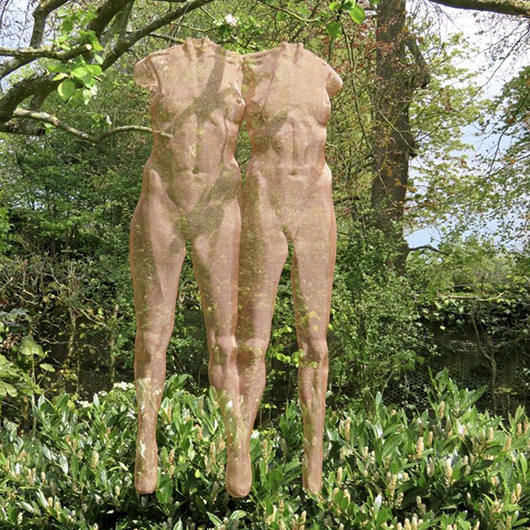Suspended double sculpture ‘TUUSUM‘, bronze mesh
 /* styles */ The Garden Gallery, Broughton, Hampshire. 18 May – 8 July ‘Making a Mark‘. We had a great day out with Charles Boyd and family 8 Northumberland Avenue, Boyds Grill & Wine Bar, who drove us to the opening event in a vintage Ford Mustang convertible! On show is ‘TUUSUM‘ at the Rachel Bebb's The Garden Gallery, Broughton, near the ancient capital city of Winchester.
 table div table+table+table+table+table+table+table+table+table+table+table+table+table div table{width:100%;padding:0}table div table+table+table+table+table+table+table+table+table+table+table+table+table div table img{width:96.23%;padding:0;float:none}table div table+table+table+table+table+table+table+table+table+table+table+table+table div table td{width:100%;padding:0 1.88% 18px}/* styles *//* styles */ An enchanting mixture of light and shade creates a fabulous setting for my two sculptures CIRCA and CIRCUM on show throughout the summer. I'm sorry we missed the Private View which drew the largest crowd ever for the event. The sculpture garden really is well worth a visit and we'll be dropping in later this summer.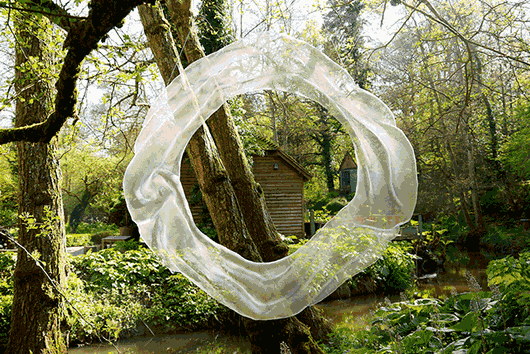‘CIRCA‘, ‘CIRCUM‘ and ‘SOLN‘ - bronze mesh sculpture
 table div table+table+table+table+table+table+table+table+table+table+table+table+table+table+table+table div table{width:100%;padding:0}table div table+table+table+table+table+table+table+table+table+table+table+table+table+table+table+table div table img{width:96.23%;padding:0;float:none}table div table+table+table+table+table+table+table+table+table+table+table+table+table+table+table+table div table td{width:100%;padding:0 1.88% 18px}/* styles */table div table+table+table+table+table+table+table+table+table+table+table+table+table+table+table+table+table+table div table{width:100%;padding:0}table div table+table+table+table+table+table+table+table+table+table+table+table+table+table+table+table+table+table div table img{width:96.23%;padding:0;float:none}table div table+table+table+table+table+table+table+table+table+table+table+table+table+table+table+table+table+table div table td{width:100%;padding:0 1.88% 18px}/* styles */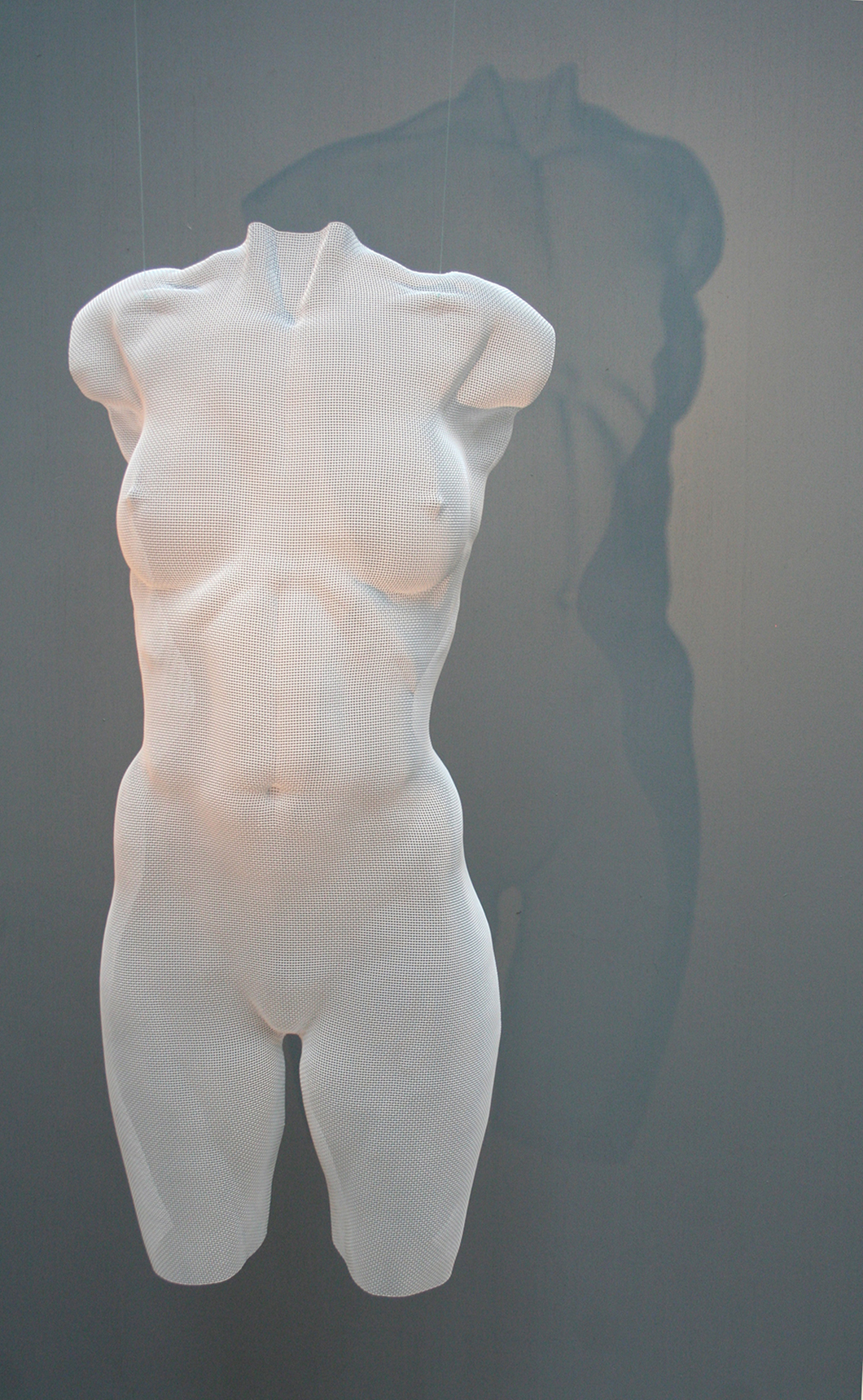‘ASPRA‘ - female torso in white painted bronzemesh will be exhibited in Munich amongst other wiremesh art.
 /* styles */ Galerie Hegemann, Munich 13th October – 18th November 2017 In this Autumn solo exhibition featuring sculpture and painting the emphasis is on the representation and use of shadows in art. I will be exhibiting torso sculpture ‘ASPRA‘ along with other new artworks. Thanks to Holger Hegemann for inviting me to exhibit in Munich once again this Autumn. More details to follow in my Autumn newsletter.
 table div table+table+table+table+table+table+table+table+table+table+table+table+table+table+table+table+table+table+table+table+table+table div table{width:100%;padding:0}table div table+table+table+table+table+table+table+table+table+table+table+table+table+table+table+table+table+table+table+table+table+table div table img{width:96.23%;padding:0;float:none}table div table+table+table+table+table+table+table+table+table+table+table+table+table+table+table+table+table+table+table+table+table+table div table td{width:100%;padding:0 1.88% 18px}/* styles */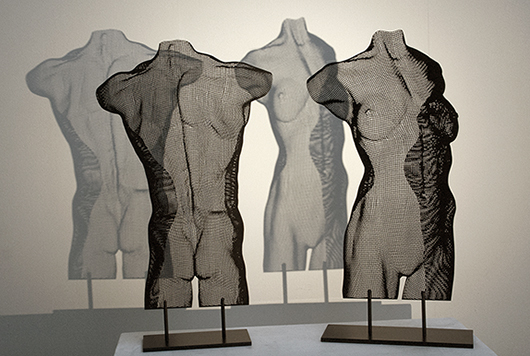Steel Panel Sculpture ‘BNUWDSTILL‘ and ‘ASTILLNUWD‘
 /* styles */ Art Byard, Cambridge 5th October – 5th November 2017 I'll be participating in ‘Shadows and Light‘, an exhibition focusing on artists who manipulate light to create dramatic effects and shadows. More news on this one too in my Autumn newsletter.
 table div table+table+table+table+table+table+table+table+table+table+table+table+table+table+table+table+table+table+table+table+table+table+table+table+table+table div table{width:100%;padding:0}table div table+table+table+table+table+table+table+table+table+table+table+table+table+table+table+table+table+table+table+table+table+table+table+table+table+table div table img{width:96.23%;padding:0;float:none}table div table+table+table+table+table+table+table+table+table+table+table+table+table+table+table+table+table+table+table+table+table+table+table+table+table+table div table td{width:100%;padding:0 1.88% 18px}/* styles */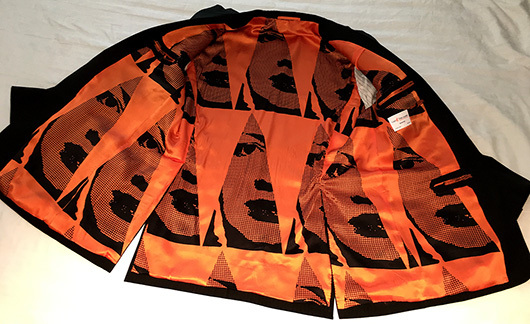Cad & the Dandy jacket lining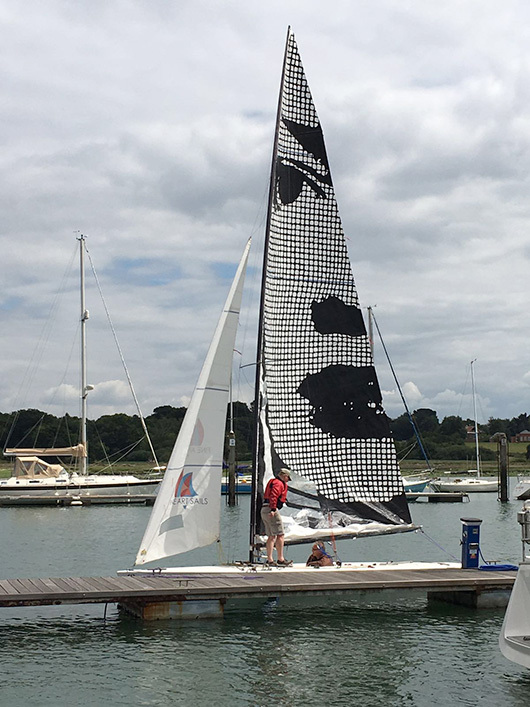‘An Eye for the Buoys‘ - Art Sail, based on steel panel sculpture ‘EVE‘

Here is the latest "eye catcher" from Michael Ross, Fine Art Sails, which features multiple images of the Olympic racing sail ‘An Eye for the Buoys‘, only this time not produced as a sail cloth but as lining for Michael Ross' unique new jacket created by Cad & the Dandy on Saville Row. I suggest he titles this one "All Eyes on Me"!

Michael is currently working on the next spectacular ArtSails event this Autumn for the Barcolana Regatta in Trieste, Italy.

 table div table+table+table+table+table+table+table+table+table+table+table+table+table+table+table+table+table+table+table+table+table+table+table+table+table+table+table+table+table div table{width:100%;padding:0}table div table+table+table+table+table+table+table+table+table+table+table+table+table+table+table+table+table+table+table+table+table+table+table+table+table+table+table+table+table div table img{width:96.23%;padding:0;float:none}table div table+table+table+table+table+table+table+table+table+table+table+table+table+table+table+table+table+table+table+table+table+table+table+table+table+table+table+table+table div table td{width:100%;padding:0 1.88% 18px}/* styles */table div table+table+table+table+table+table+table+table+table+table+table+table+table+table+table+table+table+table+table+table+table+table+table+table+table+table+table+table+table+table+table+table div table{width:100%;padding:0}table div table+table+table+table+table+table+table+table+table+table+table+table+table+table+table+table+table+table+table+table+table+table+table+table+table+table+table+table+table+table+table+table div table img{width:96.23%;padding:0;float:none}table div table+table+table+table+table+table+table+table+table+table+table+table+table+table+table+table+table+table+table+table+table+table+table+table+table+table+table+table+table+table+table+table div table td{width:100%;padding:0 1.88% 18px}/* styles */table div table+table+table+table+table+table+table+table+table+table+table+table+table+table+table+table+table+table+table+table+table+table+table+table+table+table+table+table+table+table+table+table+table+table div table{width:100%;padding:0}table div table+table+table+table+table+table+table+table+table+table+table+table+table+table+table+table+table+table+table+table+table+table+table+table+table+table+table+table+table+table+table+table+table+table div table img{width:96.23%;padding:0;float:none}table div table+table+table+table+table+table+table+table+table+table+table+table+table+table+table+table+table+table+table+table+table+table+table+table+table+table+table+table+table+table+table+table+table+table div table td{width:100%;padding:0 1.88% 18px}/* styles */